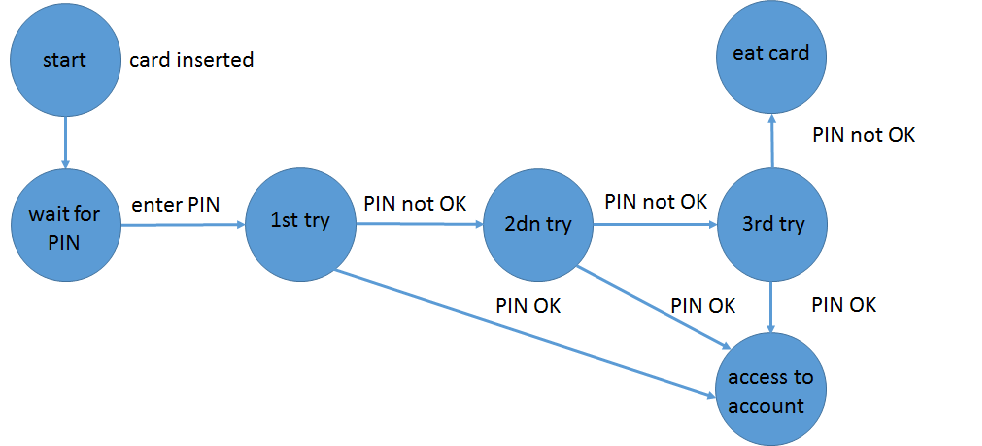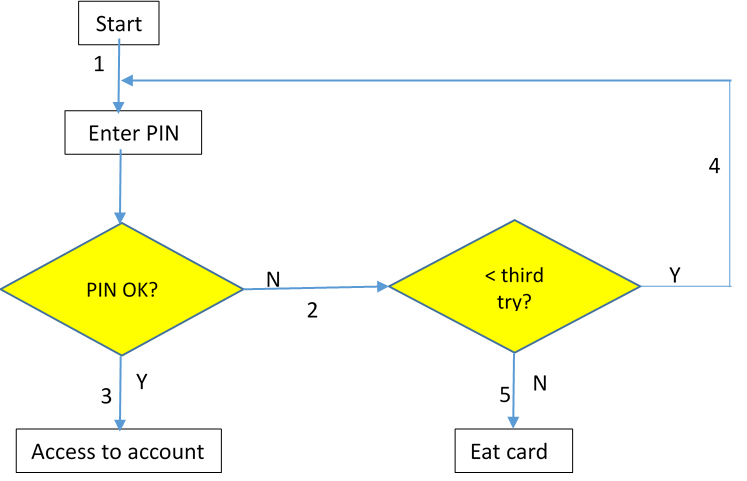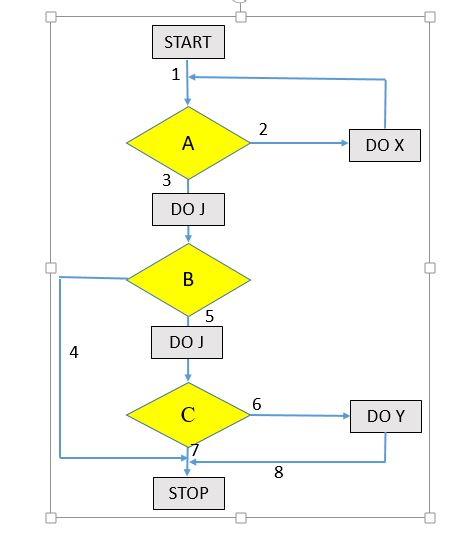# Comparison of Automated Test Design Methods

### Comparing and contrasting state transition testing, process cycle testing, and constraint-driven testing design.

· DevOps Zone · Opinion
Save
4.25K Views

The crucial part of testing is test design, which contains the creation of test cases. A test case contains preconditions, inputs and expected outputs. Test design is not an easy task, and since there are numerous methods available it is difficult to select the optimal method for any given testing process. The best methods currently available make it possible to automatically generate test cases from a special (mainly visual) representation of the specification. Usually, test design methods require a model such as UML diagrams, pseudo-code, or some special test-related model of the specification. In this article we'll compare three methods: a) State Transition Testing (STT), b) Process Cycle Testing (PCT), and c) Constraint Driven Testing (CDT).

A common advantage of these methods — besides the fact that these test design methods can be automated — is the availability of test data adequacy criteria which determine when to stop testing. In our analysis, for practical reasons we have selected the simplest adequacy criterion for each method, in which all the entities are covered once and no combination is considered. This is a realistic assumption, as most test teams generally create fewer test cases. The most efficient way to explain these three techniques is to use a common example:

## Feature: Simplified ATM

After inserting a credit card, the machine asks for a PIN code. If the PIN code is correct, then the account is accessible. If the PIN code is wrong, then the machine asks again, but after the third wrong PIN code the card will be eaten by the cash machine.

State Transition Testing (STT) is a test design method where you need to test different system transitions. It is a black box technique, which is used when the system is defined in terms of states, and the transitions between the states is managed by the rules of the system. For more details see this white paper.

The state (transition) diagram is the following:The test data adequacy criterion is to cover each edge once. An automation tool would generate four tests:

T2. (start, wait for PIN, 1st try, 2nd try, access to account)

T3. (start, wait for PIN, 1st try, 2nd try, 3rd try, access to account)

T4. (start, wait for PIN, 1st try, 2nd try, 3rd try, eat card)

An obvious problem of this technique is that the repetitive operations are tested superfluously.

Process Cycle Test (PCT), is a test design method where you need to test different processes of features, where the features are modelled by flowcharts. It is a black box technique, and can be used for any system. You can find more information here

The flowchart of the simplified ATM:PCT requires covering the following edge pairs:

• “PIN OK” decision flow line pairs: (1,2), (1,3), (4,3), (4,2)

• “< third try” decision flow line pairs: (2,4), (2,5)

To satisfy the adequacy criterion with the simplest solution an automation tool would generate the following two tests:

T1: (1,3)

T2: (1,2); (2,4); (1,2); (2,4); (1,2); (2,5);

Constraint Driven Testing (CDT) is a test design technique where categories, choices and constraints are created, based on the features of the system to be tested. Categories are the preconditions (initial state), input and sometimes the output variables. The categories are further divided into choices, which are (generalized) values. There are constraints among the choices. Read more about CDT.

Now let us consider Simplified ATM:

Categories and (choices):

• PIN code: good; wrong
• Number of entering wrong PIN code: 2; 3
• Entering another PIN code: possible; not possible

Constraints:

• IF pin code IS good, THEN Result of transaction IS access to account
• IF Number of entering wrong PIN code IS 3, THEN Result of transaction IS eat card
• IF Number of entering wrong PIN code IS 2, then Entering another PIN code IS possible
• IF Number of entering wrong PIN code IS 3, then Entering another PIN code IS not possible

Test cases are automatically generated so that each constraint is satisfied and each choice is covered at least once:

## Comparison

We originally made some assumptions based on the models behind test design methods. In this article we have assumed those test data adequacy criteria which are most reasonable for the selected test design methods. Thus for state transition testing the criterion is to cover each edge at least once. For flowchart-based models we selected a criterion of covering both of the two successive paths. Finally, for constraint driven testing we selected the all-choice, all constraint coverage criterion. These criteria are used in practice.

Since flowcharts are more compact and thus contain fewer flow lines than edges contained by state diagrams, PCT should result in fewer test cases than STT. Since (program) flowcharts represent the code to be implemented, the test cases generated by PCT will satisfy equivalence partitioning. Because of choices and constraints, CDT’s test cases will satisfy both equivalence partitioning and boundary value testing.

Since the models used by STT and PCT are code-based, these models are difficult for an agile team to create. On the contrary, CDT uses a test-based model which is easily understandable without any graphical model. It can therefore be created through teamwork, with all agile team members — including business people — able to understand and validate the model.

Even in this simple case STT results in two superfluous test cases, namely T1 and T2, since T3 covers both test cases. We can conclude that this method can be used if superfluous test cases do not cause problems, which rarely occurs.

PCT does not consider boundary value testing, so T1 is weak. Of course a manual solution would consider boundary values, but this method is considered only due to the possibility of automated test case generation. However, the main problem with any flowchart methods is that even when applying stronger test criterion, some bugs remain undetected. Consider the flowchart below:Let us assume that DO X influences DO Y, and there is a defect such that this influence is coded erroneously. Only a test involving edges 2 and 6 would reveal the fault. However, neither two successive nor three successive path criteria would be good enough to reveal the defect. On the contrary for CDT, there should be a constraint:

If DO X Then DO Y

There also has to be a test case which covers this constraint. As a consequence, the bug is detected.

We can conclude that any flowchart-based test design method with realistic testing criterion would fail for some defects. On the contrary, CDT method reveals the bugs with regards to the specification, though some non-specification related bugs may remain undetected.

Topics:
test automation, test case optimization, software testing, test optimization

Opinions expressed by DZone contributors are their own.